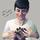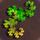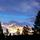## General Question# Can you help me understand this proof of countable infinity?

Asked by Mariah (25876) September 6th, 2012

My textbook is trying to show that, to prove a set is countably infinite, you can prove that the set has the same cardinality as the set of natural numbers, which is countably infinite. You prove equal cardinality by showing there is a bijection between the two sets. The example in the book is of odd positive integers.

It first proves that there is an injection like so:

“Consider the function f(n) = 2n-1” (I understand that they are defining an odd number here.)
“Suppose f(n) = f(m). Then 2n-1= 2m-1, so n=m.”

Then it proves there is a surjection:

“Suppose t is an odd positive integer. Then t is 1 less than an even integer 2k, where k is a natural number. Hence t = 2k-1 = f(k).”

I guess I’m having a hard time wrapping my mind around how either one of these proofs works. I understand what an injection and a surjection are, but something with the notation they’re using here has got my brain scrambled. Care to translate for me? I’d appreciate it a whole lot.

Observing members: 0Composing members: 0Although I have not received this level of math course yet, here is my attempt:

First, you must remember part of the definition of a function: no X value can be assigned to two or more Y values, so no two Y values can share an X value.

To prove that an infinite set is countable, then there must be a way to assign all of the members of the set to a natural number such that no two members of the set share the same natural number. This means that all countable sets are a results of functions of the natural numbers (even if the functions are transcendental).

In the second proof, the first part defines T as any odd number. It then provides a definition of the odd numbers (2K+1), where K can be any natural number. It then points out that this is a function of K, so it concludes that this is a function that can be applied to the natural numbers to generate the odd numbers. This shows that the odd numbers are countable.

The first proof is a little harder for me to understand. It may take me a minute or two to figure out, so I’ll post this answer now and then post another when I find the answer.

PhiNotPi (12677)“Great Answer” (0) Flag as…The idea is to show that there is a one-to-one mapping from the natural numbers to the odd natural numbers.
To show one-to-one (injection), suppose f(m) = f(n). Then 2m – 1 = 2n – 1 and m=n. Therefore f(m) = f(n) implies that m=n, which is the definition of one-to-one.

To show that all odd natural numbers are included in the mapping (surjection), choose any odd number t. We need to find a natural number k such that f(k) = t. Since t is odd and t >= 1, t+1 is an even number >= 2. t+1 = 2k for some natural number k. Therefore t = 2k – 1 = f(k), so every odd number is included in the mapping.

This proof is just a warmup for what is yet to come. Wait till you get to the proof that the rationals are countable and the reals are not. Although the proofs are relatively short, you get to realize what a genius Cantor was for thinking this way.

LostInParadise (28949)“Great Answer” (3) Flag as…Mariah (25876)“Great Answer” (0) Flag as…@Mariah Something else that can help with keeping these proofs straight is to think of injective as one-to-one and to think of surjective as onto. You said that you already grasped injective, so I won’t go into that one. With surjective, you’re saying that the function maps a set (it’s domain) onto (as opposed to into) another set (it’s codomain). The idea is that every element in the codomain is mapped to by some element in the domain. In other words, the codomain equals the range, or image, of the function. Hopefully that will help when you encounter these types of questions again.

bobbinhood (5896)“Great Answer” (0) Flag as…or